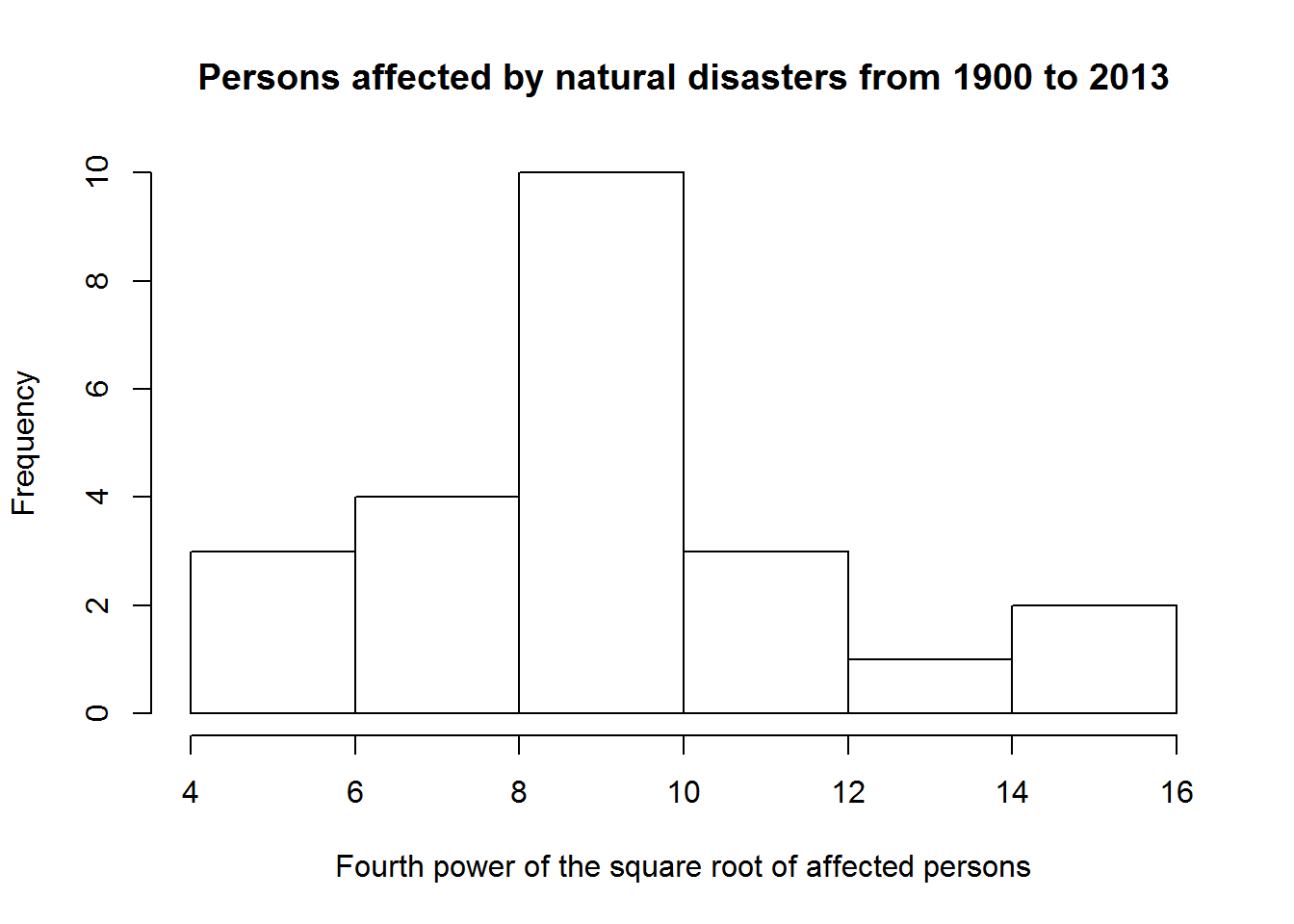BIS-Fogo

Site Tools

en:learning:schools:s01:code-examples:ba-ce-09-01

C09-1 - Generic plotting functions

The following plotting examples will show R’s generic plotting way.

The underlaying example data is taken from our combined natural disaster and Ebola data set which is loaded in the background into the data frame called df. We will generally not interpret the plots but just look at formal aspects.

Scatter plot

Scatter plots are probably the most often produced plots since they visualize the dependence between two variables in a straight forward manner.

In the following example, we will visualize the number of totally affected people against the time line. The aggregation looks like this:

dfa <- aggregate(df$total_affected, by = list(df$year), FUN = sum)
colnames(dfa) <- c("year", "total_affected")

The lattice way to produce scatter plots is using the xyplot function:

plot(dfa$year, dfa$total_affected)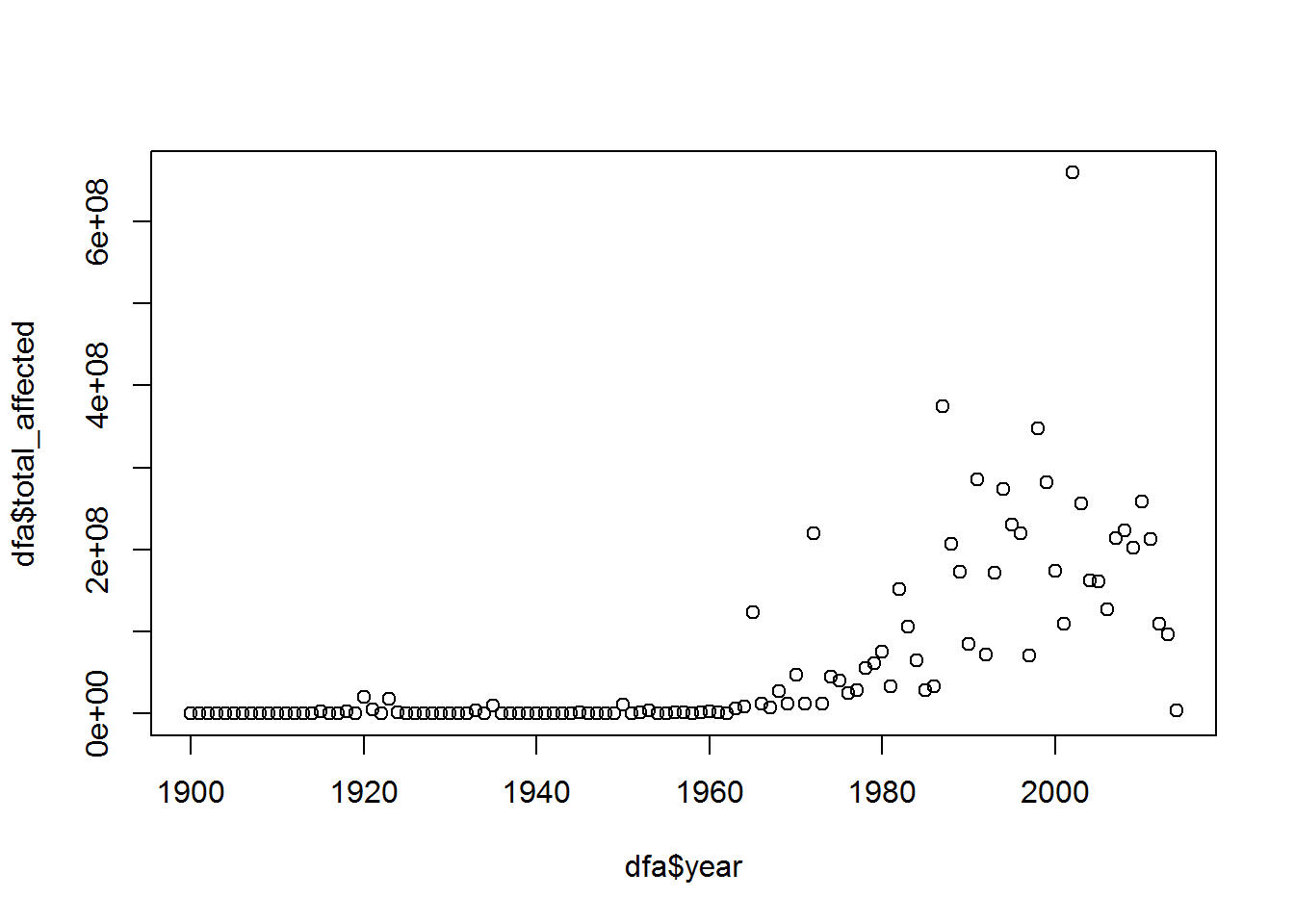The x- and y-axis still show the variable names which is a good thing if you are not sure what information is plotted on which of the axis. For presenting this plot to someone else, it might be better to add custom labels and a title.

To add custom lables, use the xlab and ylab arguments. For the title, use the main argument:

plot(dfa$year, dfa$total_affected,
xlab = "Year", ylab = "Affected persons",
main = "Persons affected by natural disasters from 1900 to 2013")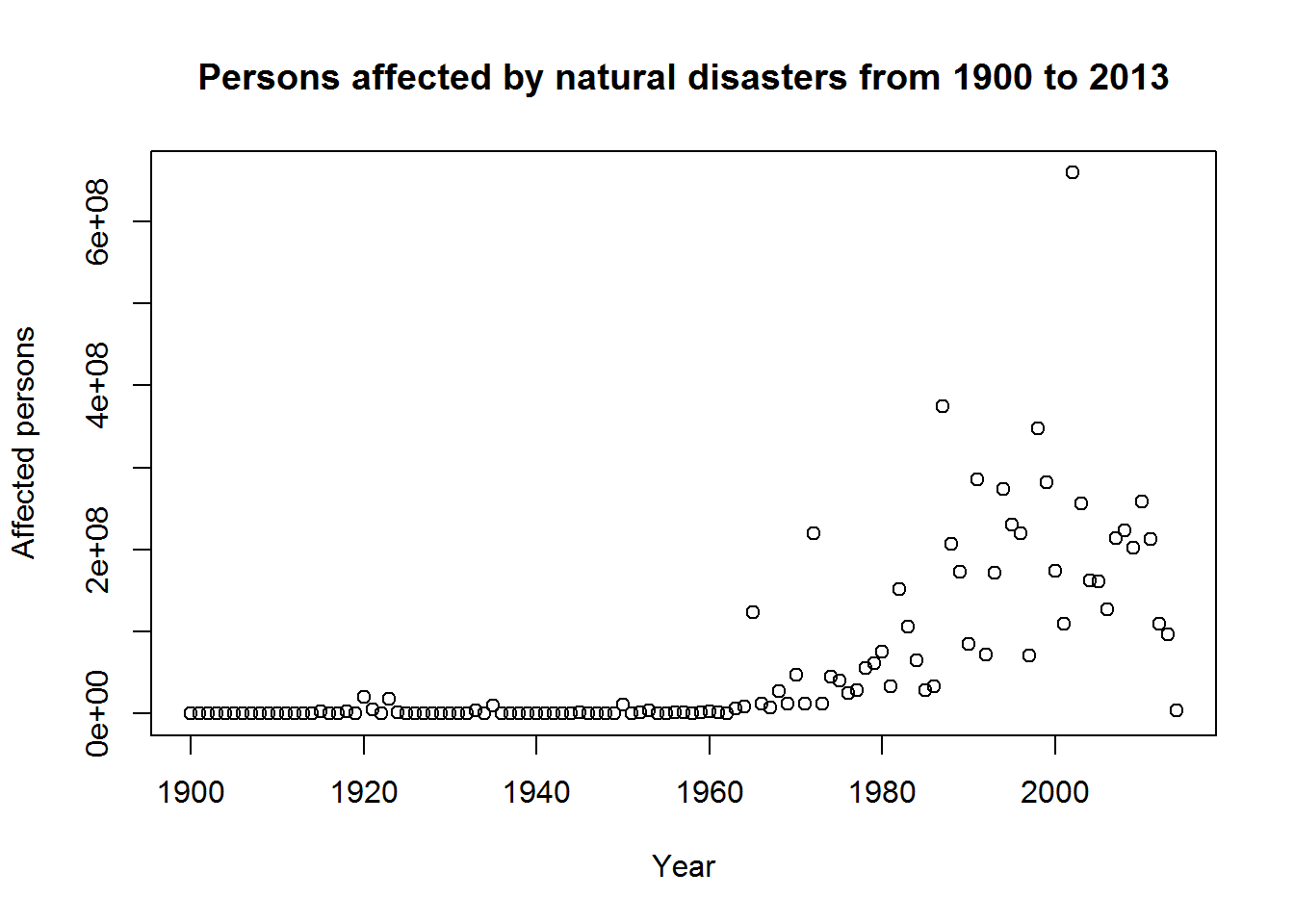If you do not like blue dots, one can of course change the color and symbols using the col and pch argument:

plot(dfa$year, dfa$total_affected,
xlab = "Year", ylab = "Affected persons",
main = "Persons affected by natural disasters from 1900 to 2013",
col = "red", pch = 13)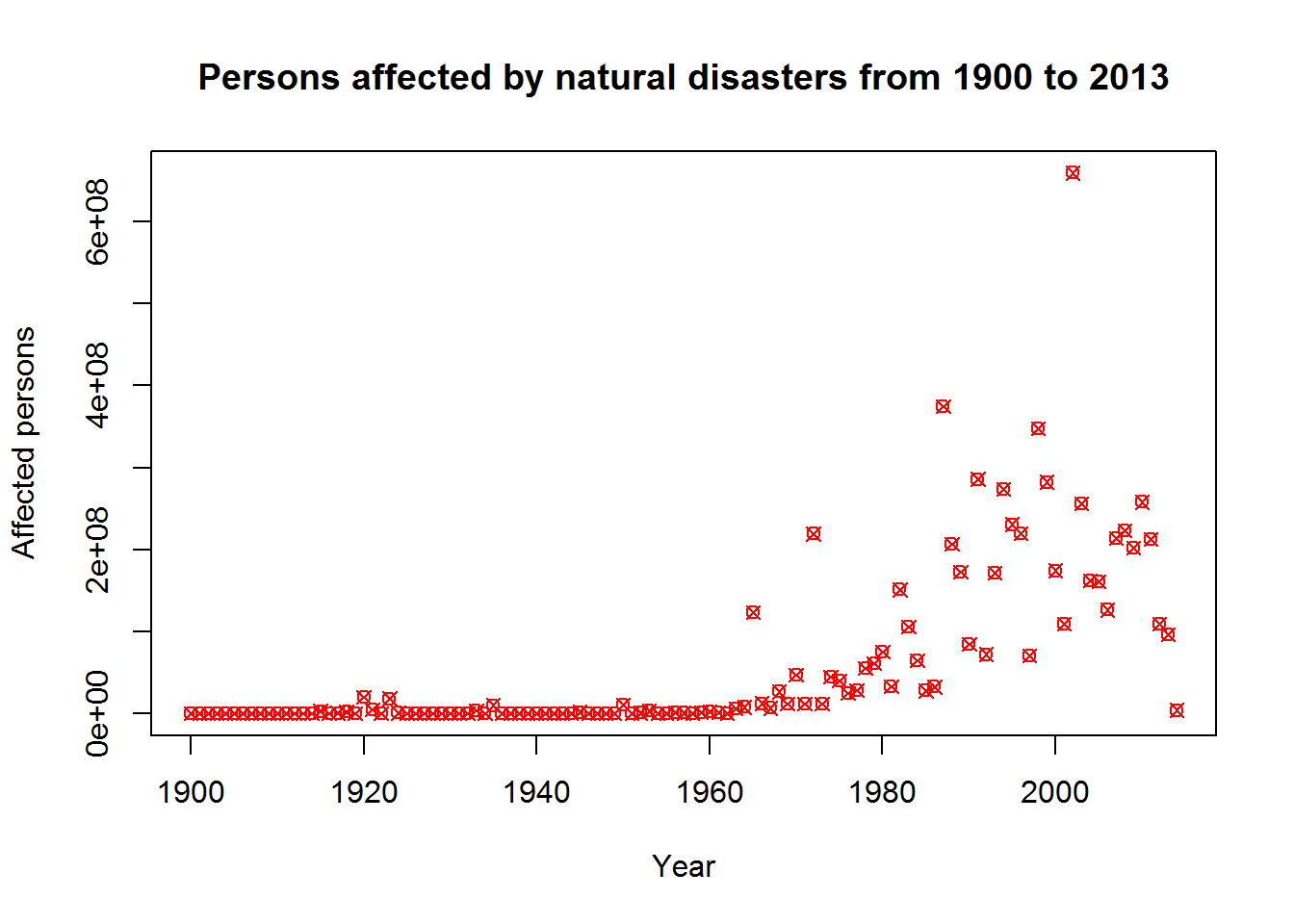And if you want filled symbols, add the bg argument (and change to a symbol which actually can be filled like the one with the number 21). To see the fill a little better, we also increase the symbol size using cex:

plot(dfa$year, dfa$total_affected,
xlab = "Year", ylab = "Affected persons",
main = "Persons affected by natural disasters from 1900 to 2013",
col = "red", bg = "blue", pch = 21, cex = 2)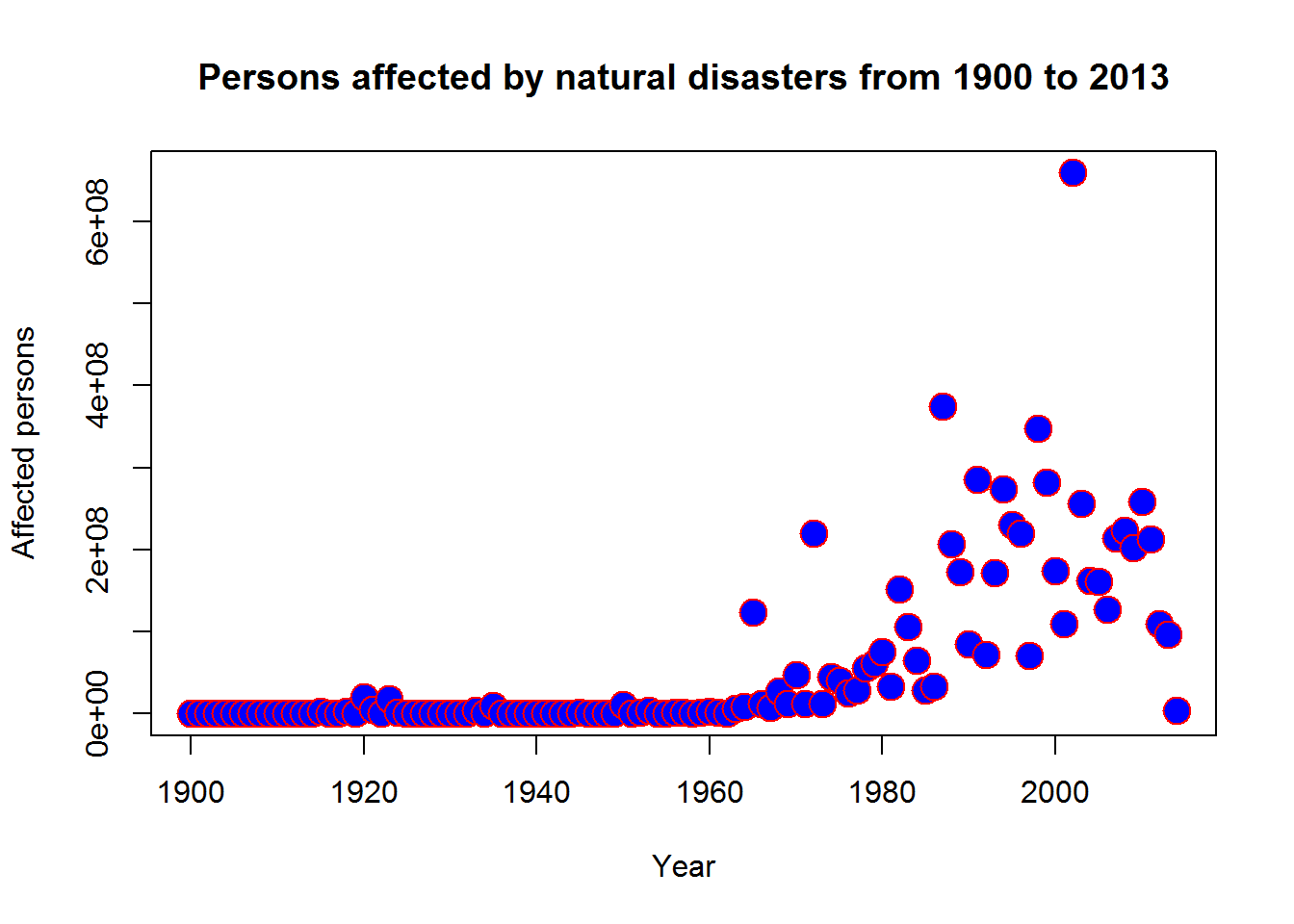Finally, a logarithmic scale on the y-axis might be of advantage to visually change the exponential increase to a more linear increase in the number of affected persons:

plot(dfa$year, dfa$total_affected,
xlab = "Year", ylab = "Affected persons",
main = "Log of persons affected by natural disasters from 1900 to 2013",
col = "red", bg = "blue", pch = 21, cex = 1,
log = "y")
## Warning: 8 y values <= 0 omitted from logarithmic plot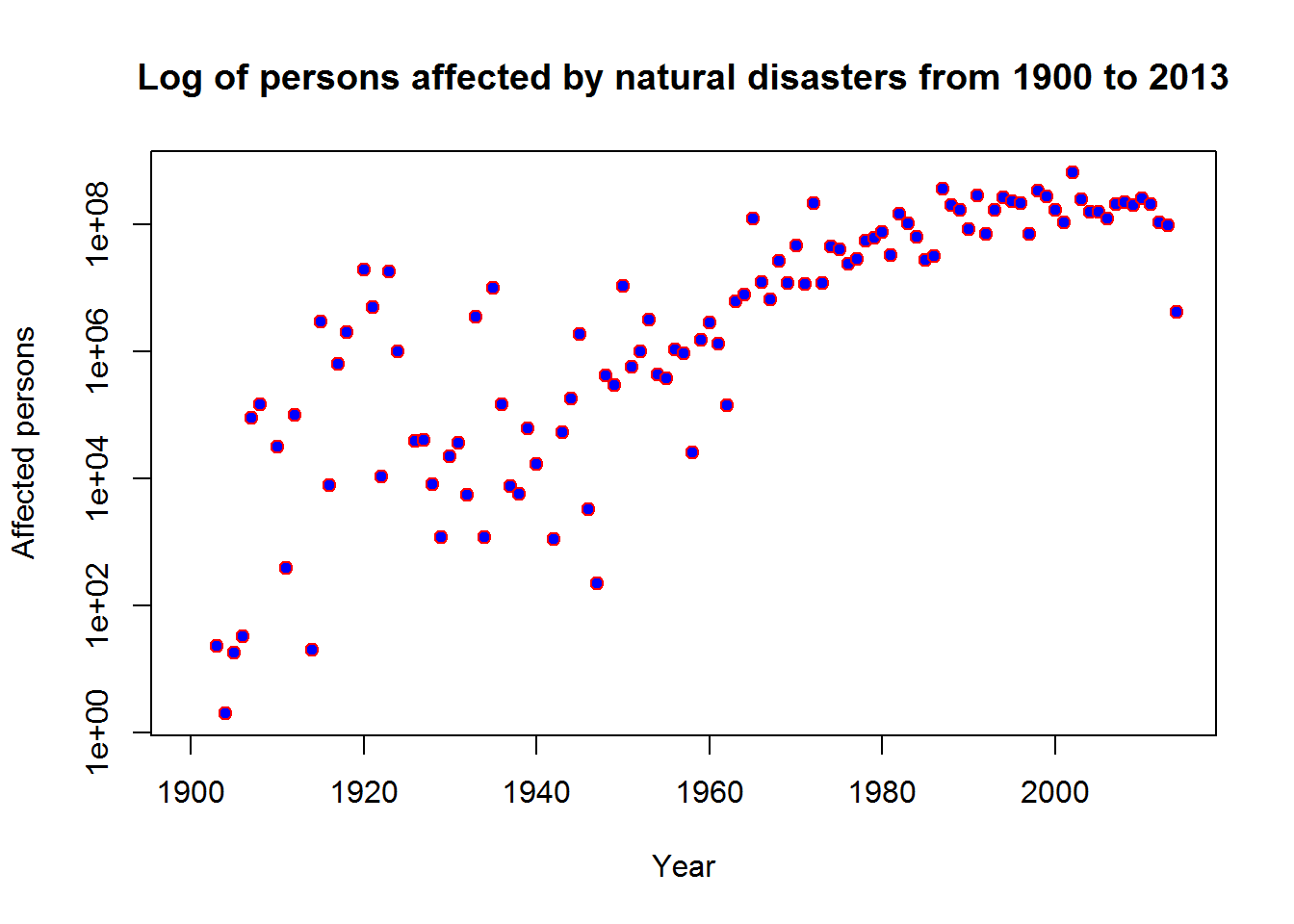Box-whisker plots

Box-whisker plots visualize some descriptive statistic values of a data set.

In the following example, we will visualize the distribution of the totally affected people across the regions. The aggregation looks like this:

dfa <- aggregate(as.numeric(df$total_affected), by = list(df$region), FUN = sum)
colnames(dfa) <- c("region", "total_affected")

To produce a box whisker plot for the variablity of the number of affected persons over all regions, use the boxplotfunction:

boxplot(dfa$total_affected, ylab = "Affected persons", main = "Persons affected by natural disasters from 1900 to 2013")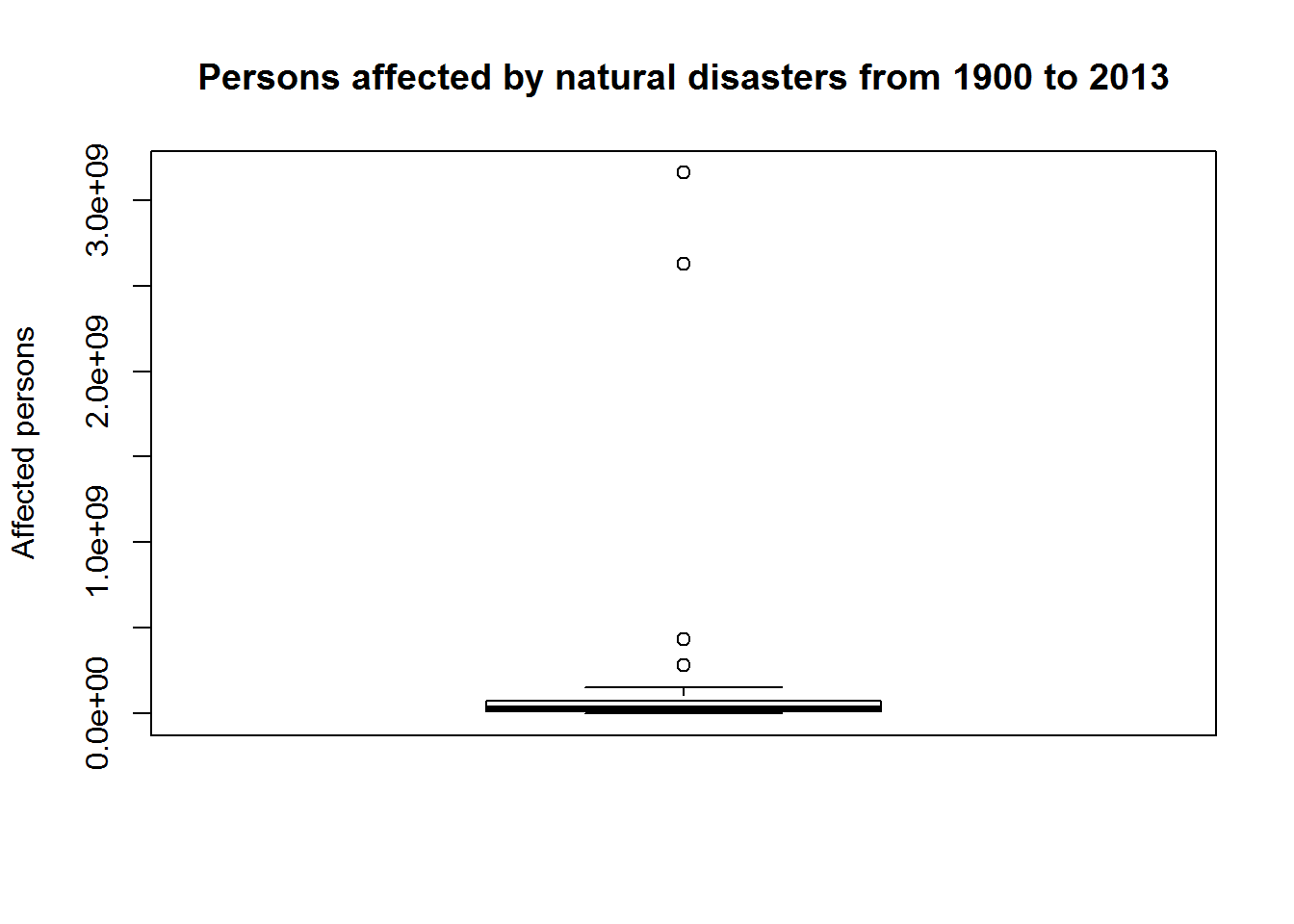As you see, you see almost nothing because of the distribution of the data values. We could use the log = “y” statement again, but a square root transformation would also be an option. Why we perform it to the third power of the root is a storyline we come back for later. boxplot((dfa$total_affected^(0.5)^3),
ylab = "Third power of the square root of affected persons",
main = "Persons affected by natural disasters from 1900 to 2013")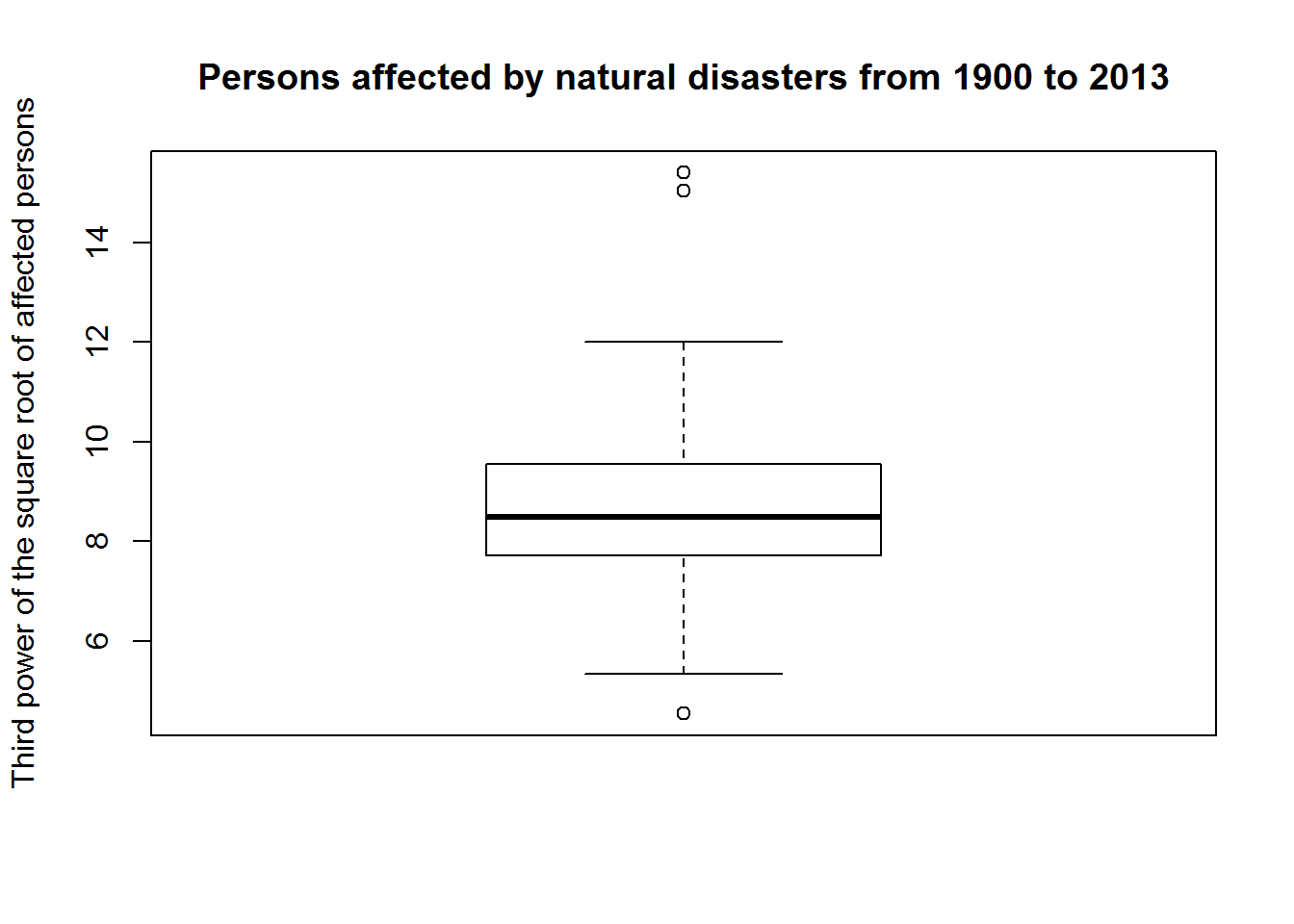The individual parts of the plot show the following:

The box includes the distribution of the values located in the second and third quartil, thus of the 50% of values which are closest to the mean value.

The median is depicted by the line in the box. The whiskers and representation of outliers represent the spread of the values.

The Whiskers mark the remaining values which don’t fall into the second and third quartile. The length of the whiskers is not standardizized. Often they are expanded to 1.5*the interquartile range (IQR).

The interquartile range is the range between the lowest value falling into the second quartile and the highest value falling into the third quartile.

All values which are higher than 1.5*IQR are considered as outliers and are usually marked by points over or under the whiskers, respectively.

One nice feature of the boxplot function is that it can also handel the aggregation of a variable. To do that, supply the aggregation variable in the function. The following example uses the original data frame and computes the distribution of the totally affected persons across all years for each region:

boxplot((total_affected^(0.5)^3) ~ region, data = df,
ylab = "Third power of the square root of affected persons",
main = "Persons affected by natural disasters from 1900 to 2013",
las=2)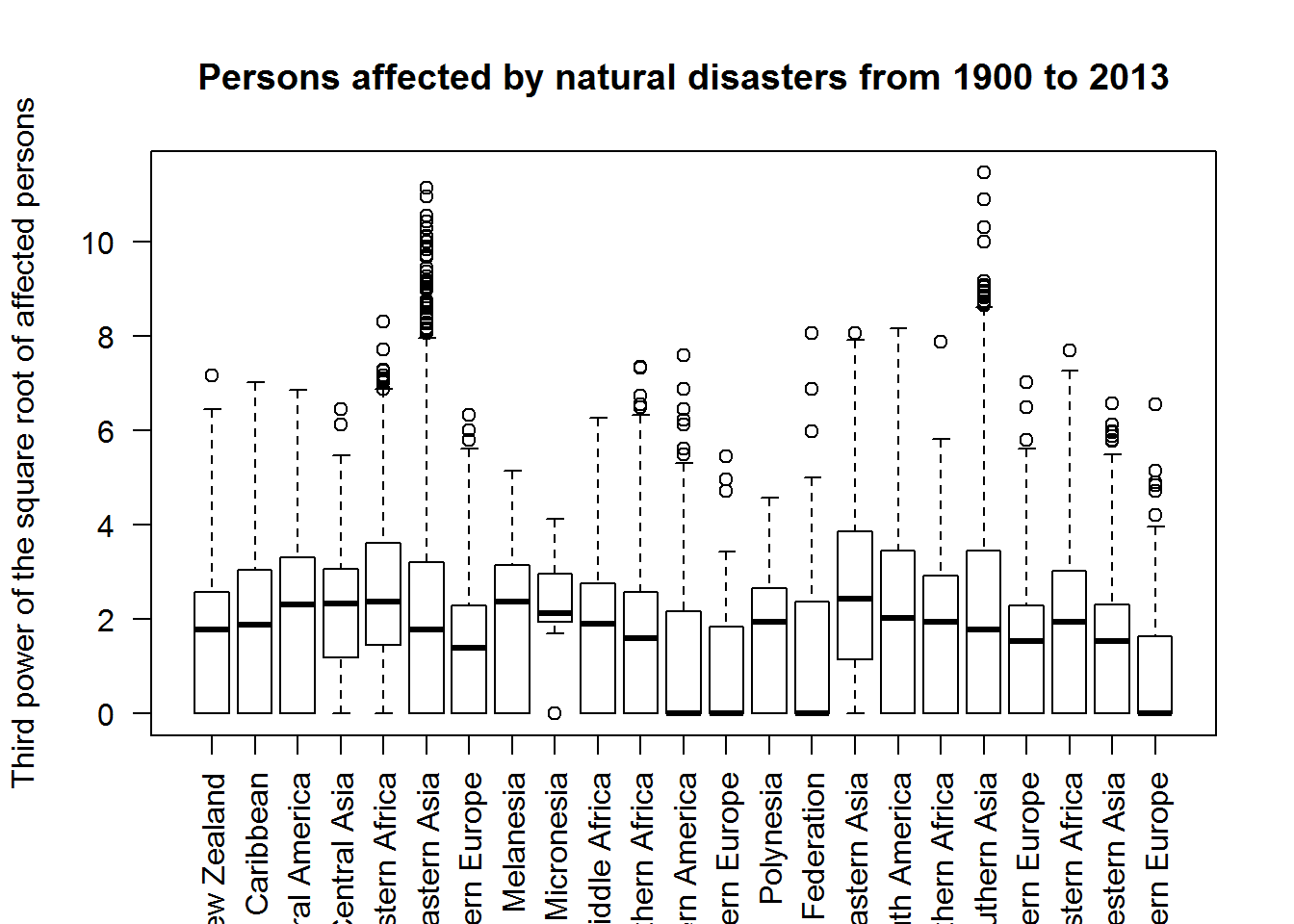The x labes are rotated by 90 degree using the las argument.

Histogramm plots

Histogramm plots show the frequency distribution of the data.

In the following example we will use the same aggregated data frame as above and have a look at the distribution of the affected persons.

A simple histogram can be compute using the hist function:

hist(dfa$total_affected, xlab = "Affected persons", main = "Persons affected by natural disasters from 1900 to 2013")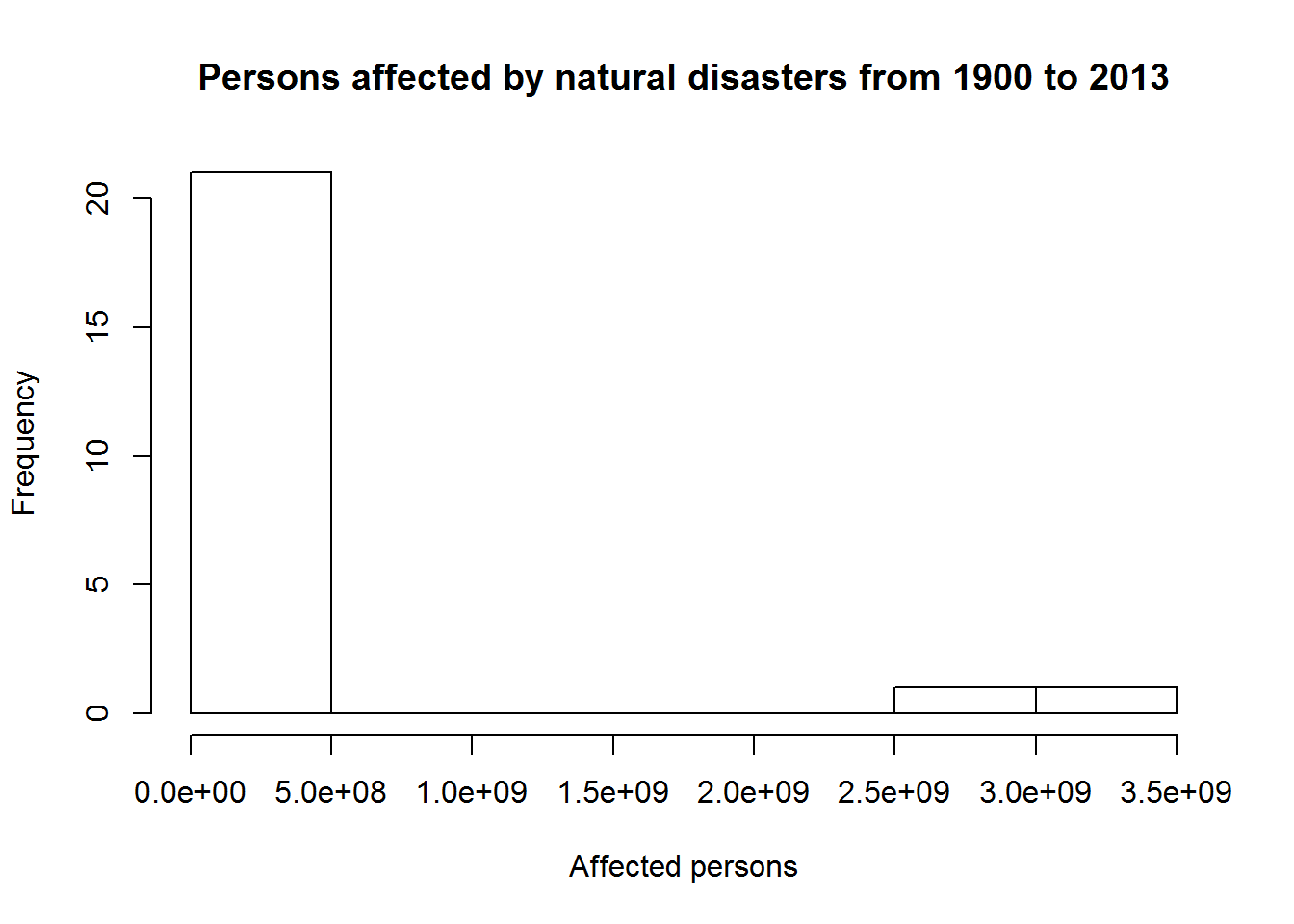As we know already from the plots above, the frequency distribution of the values is far from normaly distributed. Let’s have a look at the log transformation we used for the scatter plot: hist(log(dfa$total_affected),
xlab = "Logarithm of affected persons",
main = "Persons affected by natural disasters from 1900 to 2013")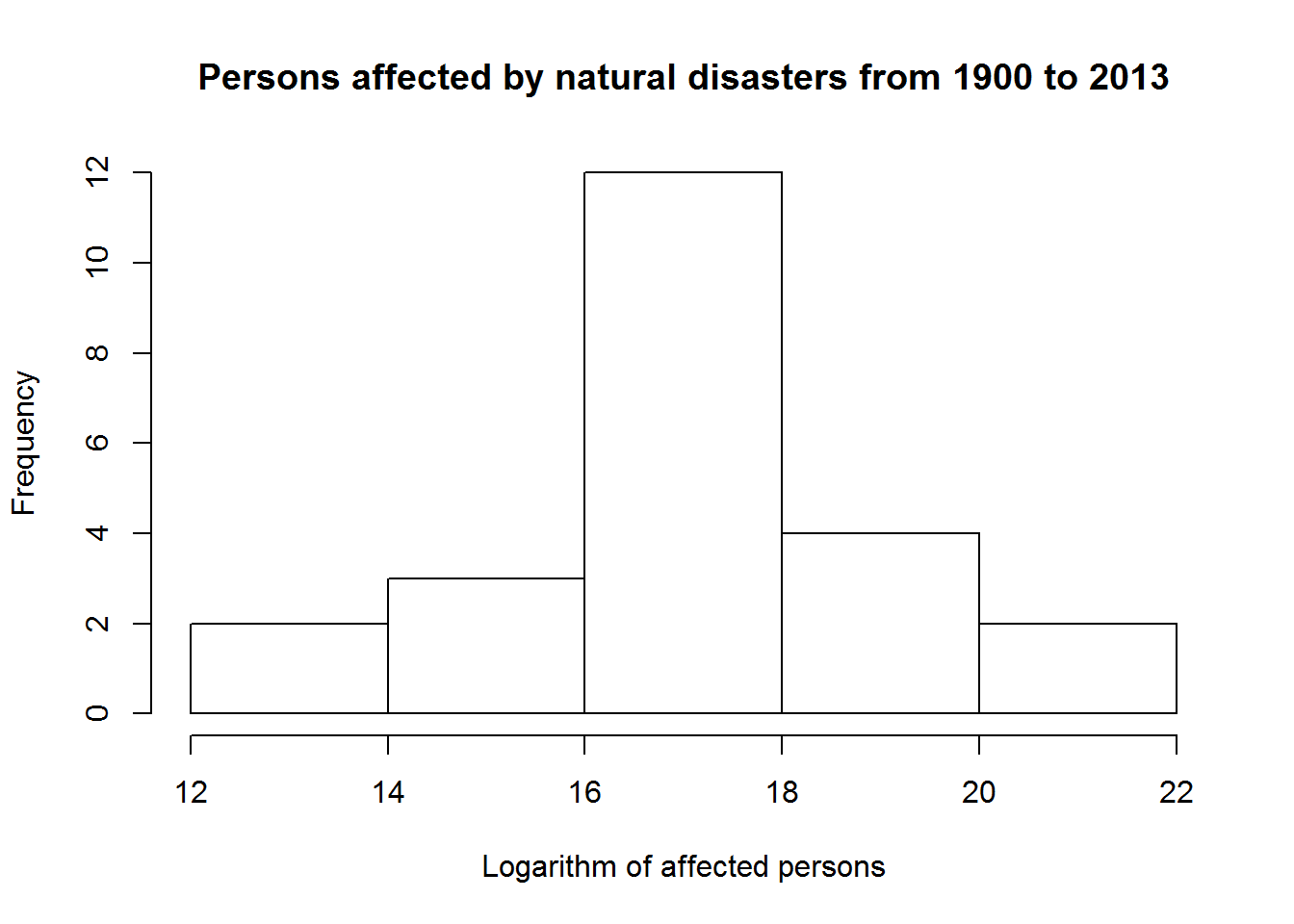It obisouly results in something quite similar to a normal distribution.

Finally, let’s check the square root transoformation used for the box whisker plots:

hist(dfa$total_affected^0.5, xlab = "Square root of affected persons", main = "Persons affected by natural disasters from 1900 to 2013")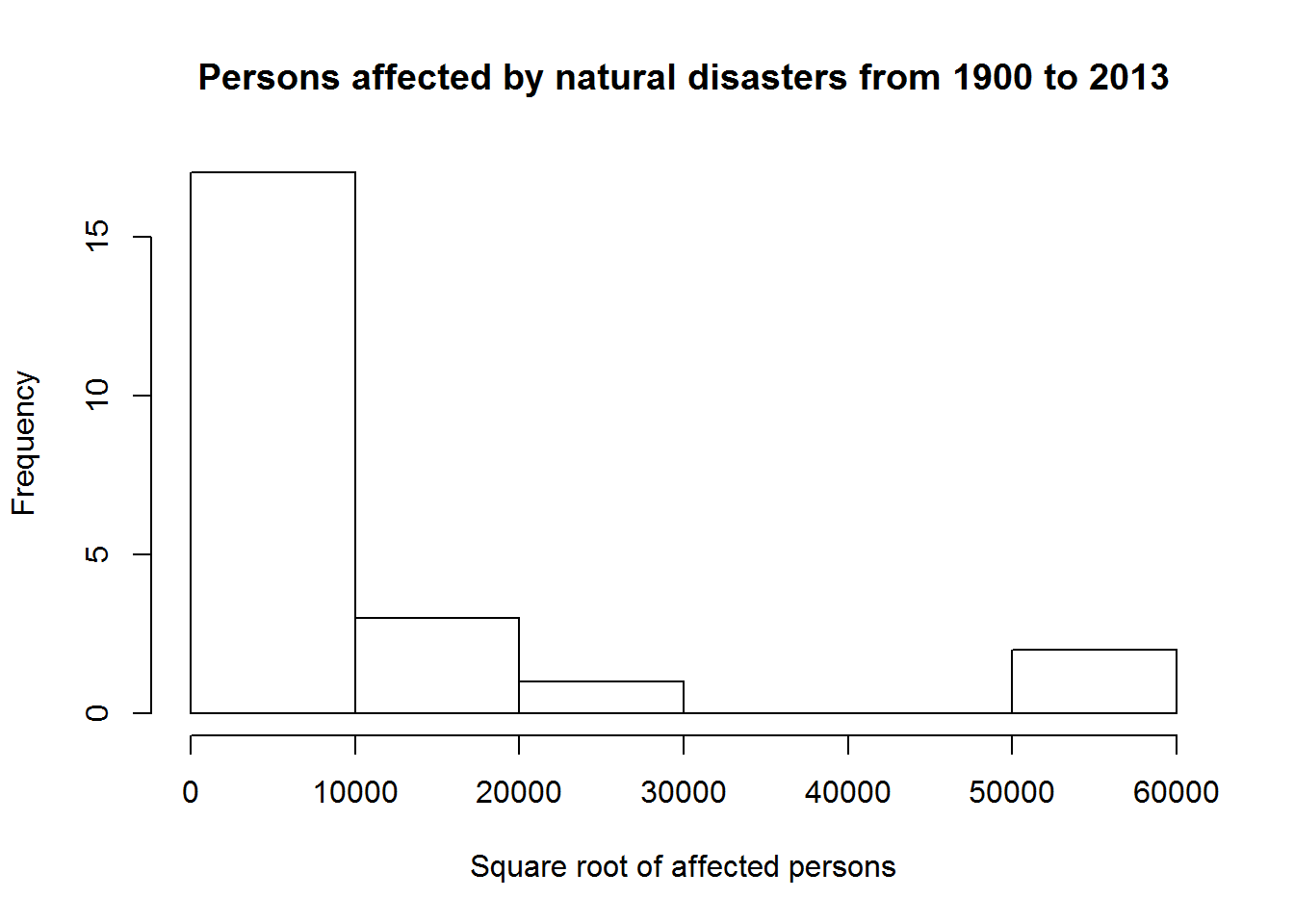That does not change much, but if we increase the power of the root, the resulting value distribution becomes more and more normaly distributed (except for the values: hist(dfa$total_affected^(0.5)^3,
xlab = "Fourth power of the square root of affected persons",
main = "Persons affected by natural disasters from 1900 to 2013")